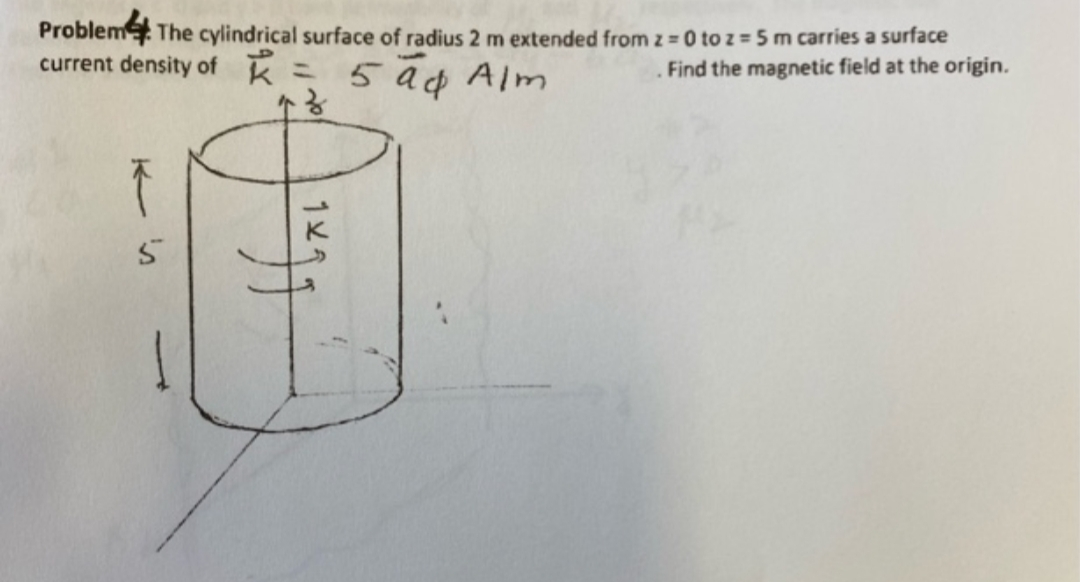# Problem The cylindrical surface of radius 2 m extended from z = 0 to z = 5 m carries a surfacecurrent density of k5 a¢ AlmFind the magnetic field at the origin.%3D

Question
14 viewshelp_outlineImage TranscriptioncloseProblem The cylindrical surface of radius 2 m extended from z = 0 to z = 5 m carries a surface current density of k 5 a¢ Alm Find the magnetic field at the origin. %3D fullscreen
check_circle

Step 1

Consider a small segment dx of circular path on the surface of cylindrical.

Calculate the differential current flowing in the in the small segment,

Step 2

Consider this segment of circular path as a ring and calculate the magnetic field at the origin.

Step 3

Integrate the above expression for z = 0 to z = 5 to calculate the total magnetic f...

### Want to see the full answer?

See Solution

#### Want to see this answer and more?

Solutions are written by subject experts who are available 24/7. Questions are typically answered within 1 hour.*

See Solution
*Response times may vary by subject and question.
Tagged in

### Electrical Engineering